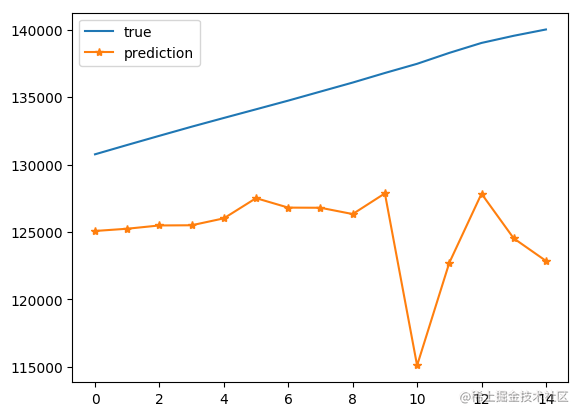# 【機器學習】LSTM神經網路實現中國人口預測（2）

theme: nico highlight: a11y-dark

# 1. 專案回顧

• 我們將根據中國人口資料集中的多個特徵（features），例如：出生人口(萬)、中國人均GPA(美元計)、中國性別比例(按照女生=100)、自然增長率(%)等8個特徵欄位，預測中國未來總人口(萬人)這1個標籤欄位。屬於多輸入，單輸出LSTM神經網路預測範疇。
• LSTM演算法是一種重要的目前使用最多的時間序列演算法，是一種特殊的RNN（Recurrent Neural Network，迴圈神經網路），能夠學習長期的依賴關係。

# 2. 資料預處理

## 2.1 構造視窗

• 因為LSTM網路是由一個個視窗進行滑動去預測的，因此，需要定義視窗函式
• 對於LSTM網路，此資料集中，“總人口(萬人)”為目標值
• 在每個視窗後的“總人口(萬人)”資料為此視窗的目標值
• 目標值為表中的第3列資料`data[ , 2]`

```python

# 視窗劃分

def split_windows(data, size): X = [] Y = [] # X作為資料，Y作為標籤 # 滑動視窗，步長為1，構造視窗化資料，每一個視窗的資料標籤是視窗末端的“總人口(萬人)” for i in range(len(data) - size): X.append(data[i:i+size, :]) Y.append(data[i+size, 2]) return np.array(X), np.array(Y) ```

## 2.2 劃分資料集

• 在訓練之前我們還需要劃分訓練集與測試集樣本
• 劃分訓練集與測試集樣本
• 切分比例為： 0.6 ：0.4
• 對劃分後的資料集進行視覺化

```python all_data = population.values

split_fraction = 0.6 train_split = int(split_fraction * int(population.shape)) # 計算切分的訓練集大小

train_data = all_data[:train_split, :] test_data = all_data[train_split:, :]

plt.figure(figsize=(10, 5))

# 資料視覺化

plt.plot(np.arange(train_data.shape), train_data[:, 2], label='train data') plt.plot(np.arange(train_data.shape, train_data.shape + test_data.shape), test_data[:, 2], label='test data') plt.legend() ``` 執行結果如下圖所示： - 資料集共有50條樣本 - 經過劃分，有30條訓練資料，20條測試資料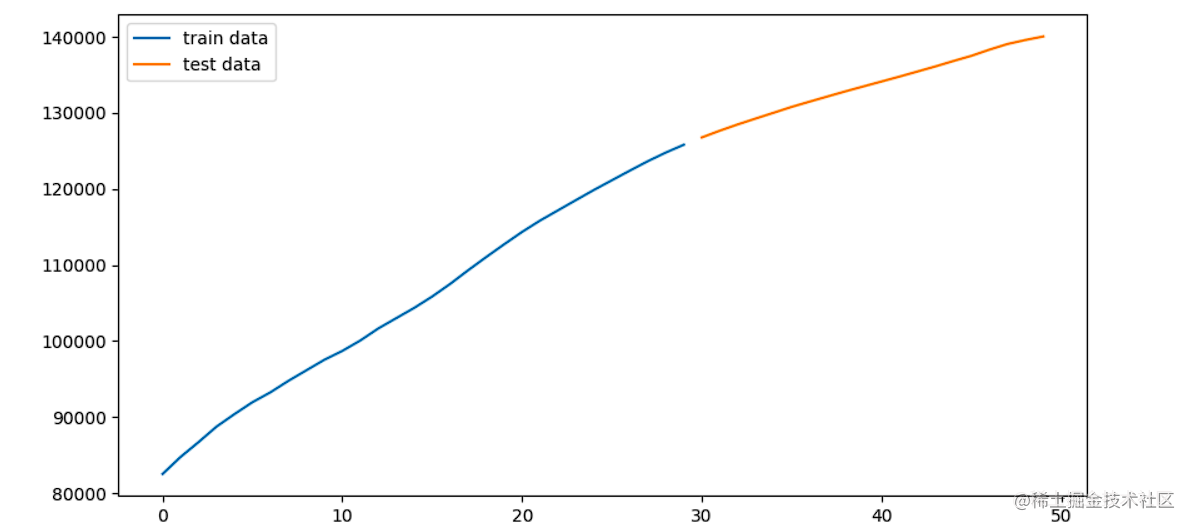## 2.3 歸一化並劃分視窗

• 使用sklearn庫中的最大最小歸一化對訓練資料與測試資料進行歸一化
• 使用之前定義的視窗劃分函式對訓練集和測試集的特徵與目標值進行劃分

```python from sklearn.preprocessing import MinMaxScaler

scaler = MinMaxScaler() scaled_train_data = scaler.fit_transform(train_data)

# 使用訓練集的最值對測試集歸一化，保證訓練集和測試集的分佈一致性

scaled_test_data = scaler.transform(test_data)

# 訓練集測試集劃分

window_size = 5 train_X, train_Y = split_windows(scaled_train_data, size=window_size) test_X, test_Y = split_windows(scaled_test_data, size=window_size) print('train shape', train_X.shape, train_Y.shape) print('test shape', test_X.shape, test_Y.shape) ``` 執行結果如下圖所示：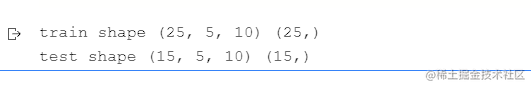```python

# 輸入的指標維度

window_size = 5 fea_num = 10 batch_size = 5 class CNN_LSTM(paddle.nn.Layer): def init(self, window_size, fea_num): super().init() self.window_size = window_size self.fea_num = fea_num self.conv1 = paddle.nn.Conv2D(in_channels=1, out_channels=64, stride=1, kernel_size=3, padding='same') self.relu1 = paddle.nn.ReLU() self.pool = paddle.nn.MaxPool2D(kernel_size=2, stride=1, padding='same') self.dropout = paddle.nn.Dropout2D(0.3)

``````    self.lstm1 = paddle.nn.LSTM(input_size=64*fea_num, hidden_size=128, num_layers=1, time_major=False)
# self.lstm2 = paddle.nn.LSTM(input_size=128, hidden_size=64, num_layers=1, time_major=False)

def forward(self, x):
x = x.reshape([x.shape, 1, self.window_size, self.fea_num])
x = self.conv1(x)
x = self.relu1(x)
x = self.pool(x)
x = self.dropout(x)

x = x.reshape([x.shape, self.window_size, -1])
x, (h, c) = self.lstm1(x)
# x, (h,c) = self.lstm2(x)
x = x[:,-1,:] # 最後一個LSTM只要視窗中最後一個特徵的輸出
x = self.fc(x)
x = self.relu2(x)

return x
``````

```

```python

# 列印網路結構

model = CNN_LSTM(window_size, fea_num) paddle.summary(model, (5, 5, 10)) # batchsize樣本數，視窗大小，特徵數量 ``` 執行結果如下圖所示：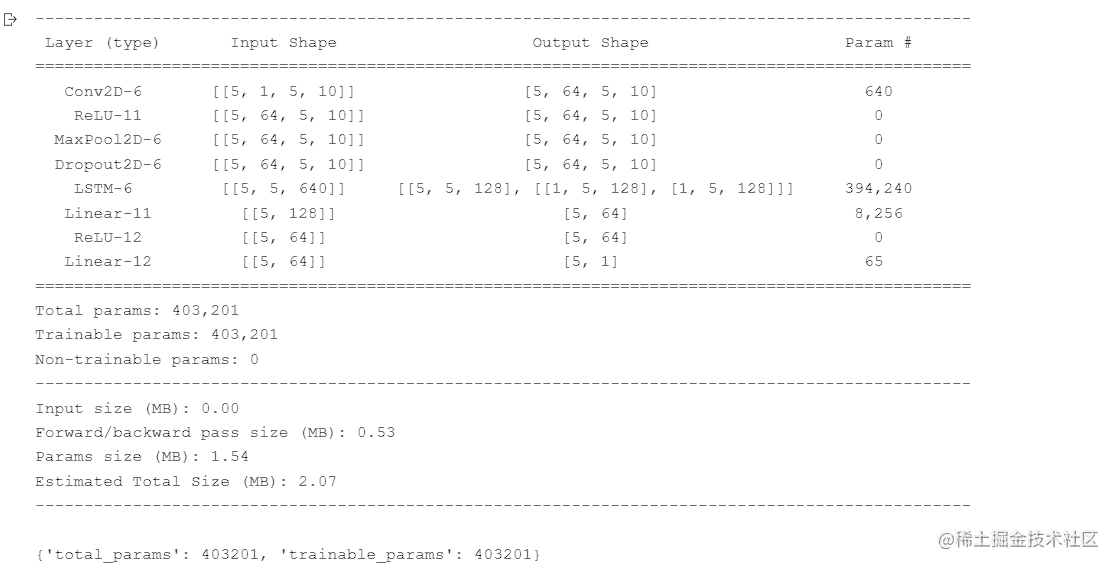# 定義超引數

base_lr = 0.005 BATCH_SIZE = 5 EPOCH = 50 lr_schedual = paddle.optimizer.lr.CosineAnnealingDecay(learning_rate=base_lr, T_max=EPOCH, verbose=True) loss_fn = nn.MSELoss() metric = paddle.metric.Accuracy() opt = paddle.optimizer.Adam(parameters=model.parameters(), learning_rate=lr_schedual, beta1=0.9, beta2=0.999) ```

```def process(data, bs): l = len(data) tmp = [] for i in range(0, l, bs): if i + bs > l: tmp.append(data[i:].tolist()) else: tmp.append(data[i:i+bs].tolist()) tmp = np.array(tmp) return tmp```

```

# 處理訓練資料

train_X = process(train_X, 5) train_Y = process(train_Y, 5) print(train_X.shape, train_Y.shape) ```

# 4. 模型訓練

• 在訓練過程中列印訓練輪數、訓練損失

• 儲存模型引數到路徑`work/cnn_lstm_ep50_lr0.005`

• 訓練超引數已在前面指定：

• base_lr = 0.005
• BATCH_SIZE = 5
• EPOCH = 50

```

# 模型訓練

for epoch in range(EPOCH): model.train() loss_train = 0

``````for batch_id, data in enumerate(train_X):
label = train_Y[batch_id]
label = label.reshape([label.shape,1])
y = model(data)

loss = loss_fn(y, label)
loss.backward()
opt.step()
loss_train += loss.item()

print("[TRAIN]epoch: {},  loss: {:.4f}".format(epoch+1, loss_train))

lr_schedual.step()
``````

# 儲存模型引數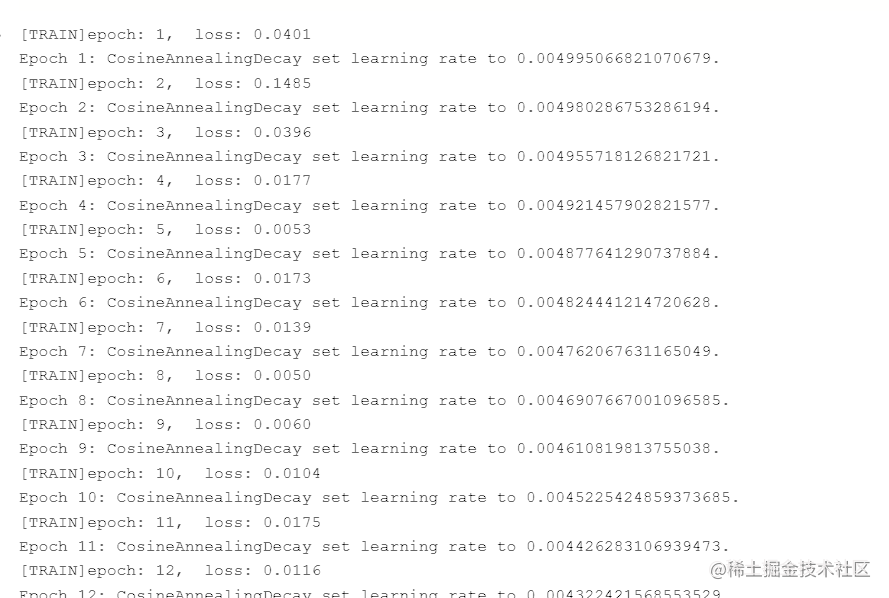```python

# 載入模型

test_X = paddle.to_tensor(test_X, dtype='float32') prediction = model(test_X) prediction = prediction.cpu().numpy() prediction = prediction.reshape(prediction.shape, )

# 反歸一化

scaled_prediction = prediction * (scaler.data_max_ - scaler.data_min_) + scaler.data_min_ scaled_true = test_Y * (scaler.data_max_ - scaler.data_min_) + scaler.data_min_

# 畫圖

plt.plot(range(len(scaled_true)), scaled_true, label='true') plt.plot(range(len(scaled_prediction)), scaled_prediction, label='prediction', marker='*') plt.legend()

``` 執行結果如下圖所示： - 經過預測，發現模型學到了資料的大體變化趨勢 - 因為本資料集樣本量太小，模型訓練後的效果有限，還需要進一步優化